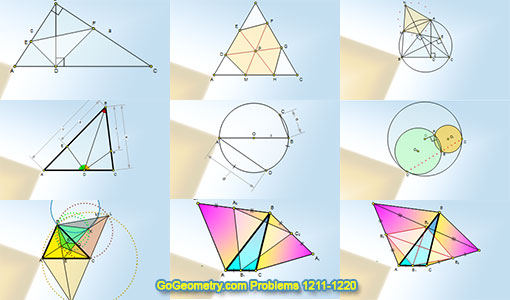# Geometry Problems 1211-1220. Level: High School, CollegeGeometry Problems 1211 - 1220Geometry Problem 1211 Right Triangle, Altitude, Angle Bisector, 45 Degrees, Metric Relations, Area. Geometry Problem 1212 Equilateral Triangle, Equilateral Hexagon, Concurrent Lines. Geometry Problem 1213 Triangle, Circumcircle, Altitudes, Cyclic Quadrilateral, Congruence. Geometry Problem 1214 Triangle, Three Angle Bisectors, Proportions. Geometry Problem 1215 Circle, Diameter, Chord, Radius, Midpoint, Measurement. Geometry Problem 1216 Circle, 90 Degrees, Tangent, Common Chord, Collinearity. Geometry Problem 1217 Triangle, Circle, Excenter, Incenter, Angle Bisector, Cyclic Quadrilateral, Circumcircle, Tangent Line. Geometry Problem 1218 Equilateral Triangles, Midpoints, 60 Degrees, Congruence. Geometry Problem 1219 Equilateral Triangles, Midpoints, 60 Degrees, Congruence, Rhombus. Geometry Problem 1220 Equilateral Triangles, Midpoints, 60 Degrees, Congruence.

Home | Sitemap | Search | Geometry | Problems | Visual Index | 10 ProblemsEmail | Post a comment | by Antonio Gutierrez
Last updated: Feb 1, 2017